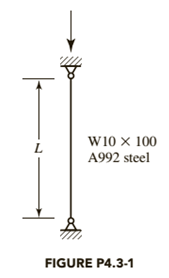Chapter 4, Problem 4.3.1P### Steel Design (Activate Learning wi...

6th Edition
Segui + 1 other
ISBN: 9781337094740

#### Solutions

Chapter
Section### Steel Design (Activate Learning wi...

6th Edition
Segui + 1 other
ISBN: 9781337094740
Textbook Problem

# Use AISC Equation E3-2 or E3-3 and determine the nominal axial compressive strength for the following cases:a. L = 10 ftb. L = 30 ftTo determine

(a)

Nominal compressive strength of column

1267.1kips

Explanation

Calculation:

Obtain the effective length factor for the support condition

Leff=L

Calculate the effective slenderness ratio using the formula as follows

Slenderness ratio =KLrConsider the properties of the steel from the AISC stell table Substitute 1 for k,(10×12)in for L,and 2.65 for ry.Slenderness ratio=KLr                           =1(10×12)2.65                             =45.2

Calculate the buckling stress by using the formula as follows:

Fe=π2E(KL/r)2

substitute 29000 for E,and 45.2 for KL/r.

Fe=π2E(KL/r)2

=π2E(KL/r)2   =π2(29000)45.22   =140.1ksi

Check for slenderness ratio by using the relation as follows:

Slenderness ratio=4.71EFyFy is the yield strength.Substitute 50ksi for Fy,and 29000 for E.Slenderness ratio=4.712900050                           =113.4Since the value 45.28 is less than 113.4, so caluculate critical buckling stress using the formula.Fcr=0.658(FyFe)Fy     =0.658(50140.1)(50)     =43.1ksi

Calculate the nominal compressive strength of column by using the formula as follows:

Pn=FcrAgSubstitute 29.40 for Ag,and 43.03 for FcrPn=FcrAg    =43.1(29.40)    =1267.1kips

Conclusion:

Therefore, the nominal compressive strength of column 1267.1kips.

To determine

(b)

Nominal compressive strength of column

400kips

Explanation

Calculation:

Calculate the effective slenderness ratio using the formula as follows

Slenderness ratio =KLrConsider the properties of the steel from the AISC steel table Substitute 1 for k,(30×12)in for L,and 2.65 for ry.Slenderness ratio=KLr                           =1(30×12)2.65                             =135.84

Calculate the buckling stress by using the formula as follows:

Fe=π2E(KL/r)2

substitute 29000 for E,and 135.84 for KL/r.

Fe=π2E(KL/r)2

=π2E(KL/r)2   =π2(29000)135.842   =15.50ksi

Check for slenderness ratio by using the relation as follows:

Slenderness ratio=4.71EFyFy is the yield strength.Substitute 50ksi for Fy,and 29000 for E.Slenderness ratio=4.712900050                           =113.4Since the value 135.84 is greater than 113.4, so caluculate critical buckling stress using the formula.Fcr=0.877Fe     =0.877(15.50)     =13.60ksi

Calculate the nominal compressive strength of column by using the formula as follows:

Pn=FerAgSubstitute 29.40 for Ag,and 13.60 for FcrPn=FcrAg    =13.60(29.40)    =400kips

Conclusion:

Therefore, the nominal compressive strength of column 400kips.

Find quality solutions to thousands of textbooks. All for just \$9.99/month

Get As ASAP

## Additional Engineering Solutions

#### Find more solutions based on key concepts